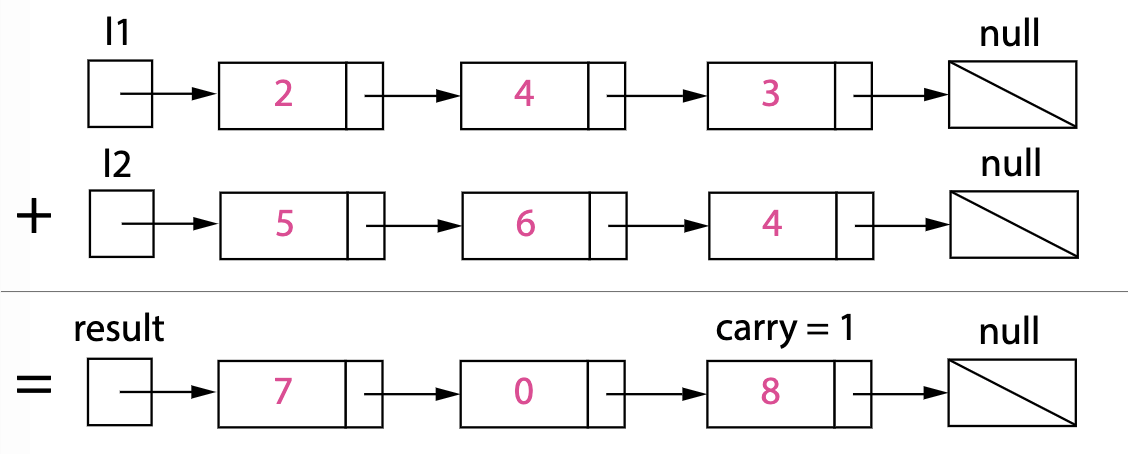Reference: LeetCode
Difficulty: Medium

## Problem

You are given two non-empty linked lists representing two non-negative integers. The digits are stored in reverse order and each of their nodes contain a single digit. Add the two numbers and return it as a linked list.

Note: You may assume the two numbers do not contain any leading zero, except the number $0$ itself.

Example:

## AnalysisThe carry must be either $0$ or $1$ because the largest possible sum of two digits is $9 + 9 + 1 = 19$.

Test Case:

### Simulation (Iteration)

Simulate digits-by-digits sum starting from the left.

Note:

• Use dummy head node of the returning list. Return dummy‘s next.
• Learn how to write better code of setting next (with null case).

Time: $O(\text{max}(M, N))$
Space: $O(\text{max}(M, N))$

### Simulation (Recursion)

Time: $O(\text{max}(M, N))$
Space: $O(\text{max}(M, N))$ including the call stack.

### Conversion

Instead of dealing with all the edge cases, just convert the linked lists to integers and convert the sum to a linked list.

Time: $O(\max(M, N))$
Space: $O(\max(M, N))$

CommentJunhao Wang
a software engineering cat